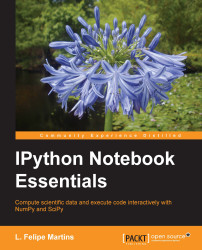•#### IPython Notebook Essentials#### Overview of this book

IPython Notebook EssentialsCreditswww.PacktPub.comPrefaceFree Chapter
A Tour of the IPython NotebookThe Notebook InterfaceGraphics with matplotlibHandling Data with pandasAdvanced Computing with SciPy, Numba, and NumbaProIPython Notebook Reference CardA Brief Review of PythonNumPy ArraysIndexWhen working with projects of some complexity, it is common to have the need to run scripts written by others. It is also always necessary to load data and save results. In this section, we will describe the facilities that IPython provides for these tasks.

### Running Python scripts

The following Python script generates a plot of a solution of the Lorenz equations, a famous example in the theory of chaos. If you are typing the code, do not type it in a cell in the notebook. Instead, use a text editor and save the file with the name `lorenz.py` in the same directory that contains the notebook file. The code is as follows:

```import numpy as np
import matplotlib.pyplot as plt
from scipy.integrate import odeint
from mpl_toolkits.mplot3d import Axes3D

def make_lorenz(sigma, r, b):
def func(statevec, t):
x, y, z = statevec
return [ sigma * (y - x),
r * x - y - x * z,
x * y - b * z ]
return func
...```